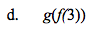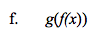### Home > CALC > Chapter 1 > Lesson 1.3.3 > Problem1-130

1-130.
1. Let f(x) = x2 and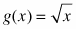. Evaluate the following expressions. Homework Help ✎

1. f(3)

2. f(−3)

3. g(9)

4. g(f(3))

5. g(f(6))

6. g(f(x))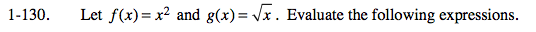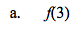f(3) = 9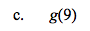Since f(x) and g(x) are inverse functions for x ≥ 0, g(9) = f(3) =______________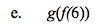Recall that f(x) and g(x) are inverse functions for x ≥ 0, and the definition of an inverse function: f(g(x)) = x.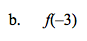Because f(x) is even, f(−3) = f(3) = part a = _____